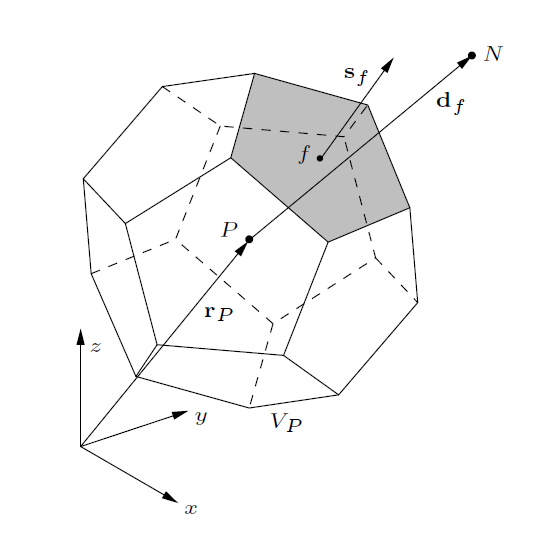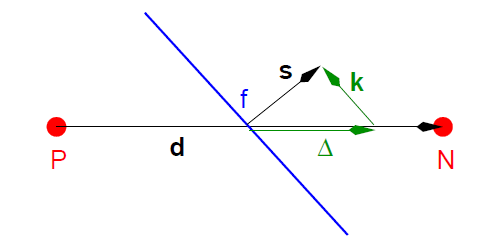## 介绍

• 基于任意多面体网格的有限体积离散方法
• 时间项的处理方式
• 对流项的处理方式
• 源项的处理方式
• 边界条件的处理方式
• OpenFOAM 中对有限体积离散的代码实现

## 基本概念

OpenFOAM 采用基于同位网格（collocated grid）的有限体积法进行离散，所有的变量都存储在控制体网格内部的同一个点上。(图片来自 Hrvoje Jasak1)

$\begin{equation} \int_{V_P} (\mathbf x - \mathbf x _P) dV = 0 \end{equation}$

$\begin{equation} \phi (\mathbf x) = \phi_P + (\mathbf x - \mathbf x_P) \cdot (\nabla \phi)_P \end{equation}$

\begin{equation} \begin{aligned} \int_{V_P} \phi (\mathbf x) dV &= \int_{V_P} [\phi_P + (\mathbf x - \mathbf x_P) \cdot (\nabla \phi)_P ] dV \\ &= \phi_P V_P + (\nabla \phi)_P \cdot \int_{V_P} (\mathbf x - \mathbf x_P) dV \\ & = \phi_P V_P \end{aligned} \end{equation}

## 对流扩散方程

$\begin{equation} \underbrace{\frac{\partial \phi}{\partial t}}_{时间项} + \underbrace{\nabla\cdot (\phi \mathbf u)}_{对流项} - \underbrace{\nabla \cdot (\gamma \nabla \phi)}_{扩散项或粘性项} = \underbrace{S_\phi(\phi)}_{源项} \end{equation}$

$\begin{equation} \int_{V_P} \frac{\partial \phi}{\partial t} dV + \int_{V_P} \nabla\cdot (\phi \mathbf u) dV - \int_{V_P} \nabla \cdot (\gamma \nabla \phi) dV = \int_{V_P} S_\phi(\phi) dV \end{equation}$

$\begin{equation} \int_{V_P} \frac{\partial \phi}{\partial t} dV + \sum_{f} [\mathbf S_f \cdot (\phi \mathbf u)_f] - \sum_{f} [\mathbf S_f \cdot (\gamma \nabla \phi)_f] = \int_{V_P} S_\phi(\phi) dV \label{eq:int_diff_conv} \end{equation}$

### 时间项的处理

$\begin{equation} \int_{V_P} \frac{\partial \phi}{\partial t} dV =\frac{\phi^n - \phi^o}{\Delta t} V_P \end{equation}$

### 对流项的处理(图片来自 OpenFOAM Foundation2)

$\begin{equation} \phi_f = \lambda \phi_P + (1 - \lambda) \phi_N \end{equation}$

$\begin{equation} \sum_{f} [\mathbf S_f \cdot (\phi \mathbf u)_f] = \underbrace{\sum_{f} [\lambda (\mathbf S_f \cdot \mathbf u_P)] \phi_P}_{P 单元} + \underbrace{\sum_{f} [(1 - \lambda) (\mathbf S_f \cdot \mathbf u_N) \phi_N]}_{与 P 相邻的所有单元} \label{eq:conv_discre} \end{equation}$

### 扩散项的处理

$\begin{equation} -\sum_{f} [\mathbf S_f \cdot (\gamma \nabla \phi)_f] = -\sum_{f} \gamma_f | \mathbf S_f | \frac{\phi_N - \phi_P}{|\overline{PN}|} \end{equation}$(图片来自 Hrvoje Jasak)

$\begin{equation} \mathbf S_f \cdot (\nabla \phi)_f = \underbrace{|\Delta| \frac{\phi_N - \phi_P}{|\overline{PN}|}}_{正交部分} + \underbrace{\mathbf k \cdot (\nabla \phi)_f}_{非正交修正部分} \end{equation}$

• 显式处理的非正交部分采用的是旧迭代步（或时间步）的值，存在信息滞后
• 显式处理的非正交部分放入源项中，将产生一个负的源项，若太大将导致求解崩溃

$\begin{equation} \mathbf k \cdot (\nabla \phi)_f < \lambda|\mathbf S_f| \frac{\phi_N - \phi_P}{|\overline{PN}|} \end{equation}$

### 源项的处理

$\begin{equation} S_\phi(\phi) = Su (\phi) + Sp (\phi) \phi \end{equation}$

$\eqref{eq:int_diff_conv}$ 中的源项可以写作

$\begin{equation} \int_{V_P}S_\phi (\phi) dV = Su V_P + Sp V_P \phi_P \label{eq:linearized_source_term} \end{equation}$

$\begin{equation} a_P \phi_P + \sum_f a_N \phi_N = r_P \end{equation}$

$\begin{equation} \mathbf A \pmb \phi = \mathbf R \label{eq:discre_coeffs_matrix} \end{equation}$

$\mathbf A$ 被称为系数矩阵 ，通常被分为三部分，分别是下三角元素 $\mathbf L$ 、对角元素 $\mathbf D$ 和上三角元素 $\mathbf U$ 。

### 边界条件的处理

• 对流项

$\begin{equation} \mathbf S_f \cdot (\phi \mathbf u)_f = \mathbf S_b \cdot (\phi \mathbf u)_b \end{equation}$

• 扩散项

$\begin{equation} \mathbf S_f \cdot (\gamma \nabla \phi)_f = \mathbf S_b \cdot (\gamma \nabla \phi)_b = \gamma_b |\mathbf S_b |\frac{\phi_b - \phi_P}{\mathbf d_b} \end{equation}$

$\phi_P$ 影响 $\mathbf D$，而 $\phi_b$ 影响 $\mathbf R$ 。

• 对流项

$\begin{equation} \mathbf S_f \cdot (\phi \mathbf u)_f = \mathbf S_b \cdot \mathbf u_b (\phi_P + |\mathbf d_b| g_b) \end{equation}$

• 扩散项

$\begin{equation} \mathbf S_f \cdot (\gamma \nabla \phi)_f = \gamma_b |\mathbf S_f| g_b \end{equation}$

## 代码实现

### 系数矩阵

Foam::lduMatrix<Type>::diag()
Foam::lduMatrix<Type>::lower()
Foam::lduMatrix<Type>::upper()
Foam::fvMatrix<Type>::D()
Foam::fvMatrix<Type>::A()
Foam::fvMatrix<Type>::source()
• lower() 返回下三角元素 $\mathbf L$

• diag() 返回不是对角元素 $\mathbf D$，而是未考虑边界条件影响的对角元素

• upper() 返回上三角元素 $\mathbf U$

• D() 返回的是对角元素 $\mathbf D$，通过 addCmptAvBoundaryDiag 施加边界条件影响

 1 2 3 4 5 6 7 8  // OpenFOAM-dev/src/finiteVolume/fvMatrices/fvMatrix/fvMatrix.C#L703 template Foam::tmp Foam::fvMatrix::D() const { tmp tdiag(new scalarField(diag())); addCmptAvBoundaryDiag(tdiag.ref()); return tdiag; } 
• A() 返回的不是 $\eqref{eq:discre_coeffs_matrix}$ 中的 $\mathbf A$ ，是 $\mathbf D$ 除以网格体积之后的值。
  1 2 3 4 5 6 7 8 9 10 11 12 13 14 15 16 17 18 19 20 21 22 23 24 25 26 27  // OpenFOAM-dev/src/finiteVolume/fvMatrices/fvMatrix/fvMatrix.C#L736 template Foam::tmp Foam::fvMatrix::A() const { tmp tAphi ( new volScalarField ( IOobject ( "A("+psi_.name()+')', psi_.instance(), psi_.mesh(), IOobject::NO_READ, IOobject::NO_WRITE ), psi_.mesh(), dimensions_/psi_.dimensions()/dimVol, extrapolatedCalculatedFvPatchScalarField::typeName ) ); tAphi.ref().primitiveFieldRef() = D()/psi_.mesh().V(); tAphi.ref().correctBoundaryConditions(); return tAphi; } 

### 时间项

  1 2 3 4 5 6 7 8 9 10 11 12 13 14  // OpenFOAM-dev/src/finiteVolume/finiteVolume/fvm/fvmDdt.C template tmp> ddt ( const GeometricField& vf ) { return fv::ddtScheme::New ( vf.mesh(), vf.mesh().ddtScheme("ddt(" + vf.name() + ')') ).ref().fvmDdt(vf); } 

### 对流项

  1 2 3 4 5 6 7 8 9 10 11  // OpenFOAM-dev/src/finiteVolume/finiteVolume/fvm/fvmDiv.C#L75 template tmp> div ( const surfaceScalarField& flux, const GeometricField& vf ) { return fvm::div(flux, vf, "div("+flux.name()+','+vf.name()+')'); } 

  1 2 3 4 5 6 7 8 9 10 11 12 13 14 15 16 17  // OpenFOAM-dev/src/finiteVolume/finiteVolume/fvm/fvmDiv.C#L43 template tmp> div ( const surfaceScalarField& flux, const GeometricField& vf, const word& name ) { return fv::convectionScheme::New ( vf.mesh(), flux, vf.mesh().divScheme(name) )().fvmDiv(flux, vf); } 

### 扩散项

  1 2 3 4 5 6 7 8 9 10 11 12 13 14 15 16 17 18 19 20 21 22 23 24  // OpenFOAM-dev/src/finiteVolume/finiteVolume/fvm/fvmLaplacian.C#L179 template tmp> laplacian ( const dimensioned& gamma, const GeometricField& vf ) { const GeometricField Gamma ( IOobject ( gamma.name(), vf.instance(), vf.mesh(), IOobject::NO_READ ), vf.mesh(), gamma ); return fvm::laplacian(Gamma, vf); } 

  1 2 3 4 5 6 7 8 9 10 11 12 13 14 15 16  // OpenFOAM-dev/src/finiteVolume/finiteVolume/fvm/fvmLaplacian.C#L206 template tmp> laplacian ( const GeometricField& gamma, const GeometricField& vf, const word& name ) { return fv::laplacianScheme::New ( vf.mesh(), vf.mesh().laplacianScheme(name) ).ref().fvmLaplacian(gamma, vf); } 

### 源项

fvm::susu 的值乘以网格体积后施加到 source() 上：

  1 2 3 4 5 6 7 8 9 10 11 12 13 14 15 16 17 18 19 20 21 22 23 24 25  // OpenFOAM/src/finiteVolume/finiteVolume/fvm/fvmSup.C#L32 template Foam::tmp> Foam::fvm::Su ( const DimensionedField& su, const GeometricField& vf ) { const fvMesh& mesh = vf.mesh(); tmp> tfvm ( new fvMatrix ( vf, dimVol*su.dimensions() ) ); fvMatrix& fvm = tfvm.ref(); fvm.source() -= mesh.V()*su.field(); return tfvm; } 

fvm::spsp 的值乘以网格体积后施加到对角元素 diag() 上：

  1 2 3 4 5 6 7 8 9 10 11 12 13 14 15 16 17 18 19 20 21 22 23 24 25  // OpenFOAM/src/finiteVolume/finiteVolume/fvm/fvmSup.C#L98 templatetemplate Foam::tmp> Foam::fvm::Sp ( const volScalarField::Internal& sp, const GeometricField& vf ) { const fvMesh& mesh = vf.mesh(); tmp> tfvm ( new fvMatrix ( vf, dimVol*sp.dimensions()*vf.dimensions() ) ); fvMatrix& fvm = tfvm.ref(); fvm.diag() += mesh.V()*sp.field(); return tfvm; } 

fvm::susp 较复杂，它为了数值稳定性考虑，把源项里的正负成分（对应源和汇）分开处理，将大于 0 的部分乘以网格体积后施加到对角元素 diag() 上，小于 0 的部分乘以网格体积和 $\phi_P^o$ 后施加到 source() 上：

  1 2 3 4 5 6 7 8 9 10 11 12 13 14 15 16 17 18 19 20 21 22 23 24 25 26 27 28  // OpenFOAM/src/finiteVolume/finiteVolume/fvm/fvmSup.C#L190 template Foam::tmp> Foam::fvm::SuSp ( const volScalarField::Internal& susp, const GeometricField& vf ) { const fvMesh& mesh = vf.mesh(); tmp> tfvm ( new fvMatrix ( vf, dimVol*susp.dimensions()*vf.dimensions() ) ); fvMatrix& fvm = tfvm.ref(); fvm.diag() += mesh.V()*max(susp.field(), scalar(0)); fvm.source() -= mesh.V()*min(susp.field(), scalar(0)) *vf.primitiveField(); return tfvm; } 

## 参考资料

1. Jasak, H. (2009). Finite Volume Discretisation with Polyhedral Cell Support. [return]
2. OpenFOAM Foundation (2015). OpenFOAM Programmers' Guide Version 3.0.0. [return]